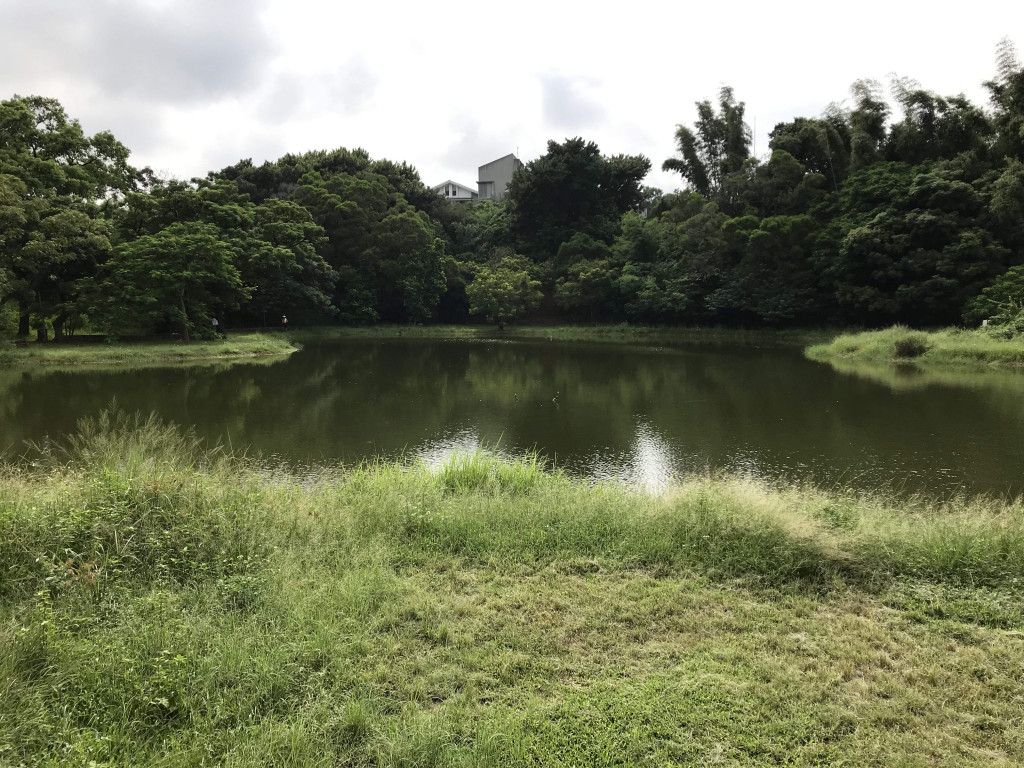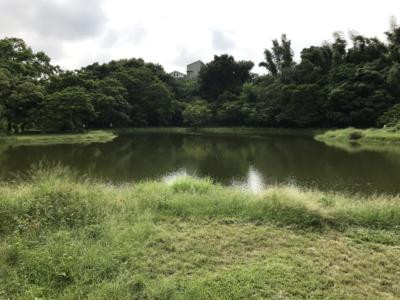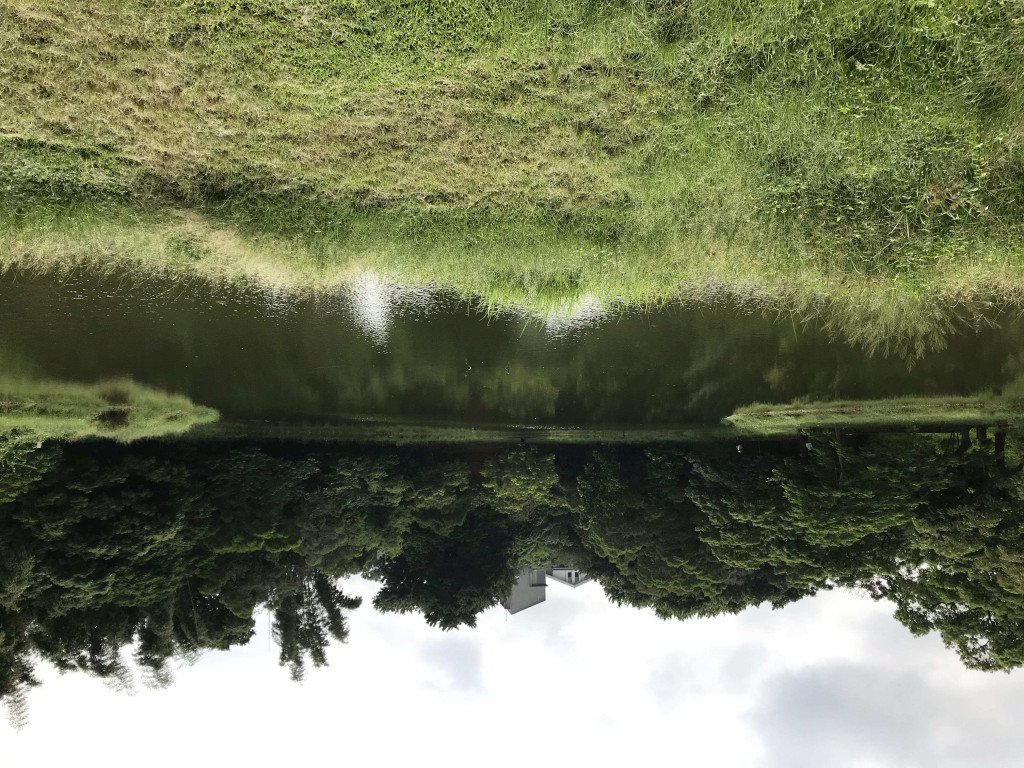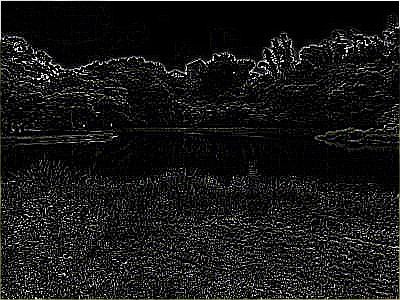#DAY 24
0

## Day24-Python 影像處理套件 PIL

PIL(Pillow) 是 Python 中著名的影像處理套件，以前在 Python2 的時代是 PIL，到 Python3 fork 出一個可相容新版的 Pillow，可以用來轉檔、調色、濾鏡、浮水印甚至創造圖片一堆的功能，雖然不能像 photoshop 之類的軟體一樣強大，但他可以透過程式語言批次處理大量的檔案，在其他需要用到圖片的應用也有很大的幫助。

### 安裝

``````pipenv --python 3.7
pipenv shell
pipenv install Pillow
``````

### 轉檔

`Image.open()` 讀取圖片檔
`save("FILE NAME","FORMAT")` 用來寫入圖片檔，format 其實非必須，PIL會自動透過常見副檔名來判斷格式。

``````from PIL import Image

im = Image.open("test.jpg")
im.save("test.png","png")
``````
``````from PIL import Image

im = Image.open("test.jpg")
im.save("test.tiff","tiff")
``````
``````from PIL import Image

im = Image.open("test.jpg")
im.save("test.pdf","pdf")
``````

• BMP
• EPS
• GIF
• JPEG
• PNG
• TIFF
• PDF

### 將目錄下所有 jpg 檔轉換為特定格式

Usage: python convert.py [Format]
e.g. python convert.py png

``````from glob import glob
from os.path import splitext
from PIL import Image
import sys

if(len(sys.argv) != 2):
exit("參數錯誤")

userFormat = sys.argv

jpglist = glob("*.[jJ][pP][gG]")

for jpg in jpglist:
try:
im = Image.open(jpg)
pic = splitext(jpg) + "." + userFormat
im.save(pic)
print(pic)
except expression as identifier:
print(identifier)
``````

### 修改突變尺寸

``````from PIL import Image

im = Image.open("test.jpg")
print (im.size)
width = 400
ratio = float(width)/im.size
height = int(im.size*ratio)
nim = im.resize( (width, height), Image.BILINEAR )
print (nim.size)
nim.save("test_resized.jpg")
``````### JPG 壓縮

`save()`函式中加入 `quality=80`, `subsampling=0`這兩個參數即可壓縮檔案，通常 quality 會設定80或90。

``````from PIL import Image

img = Image.open("test.jpg")
img.save("test_c.jpg",quality=65, subsampling=0)
``````

### 圖片旋轉

• Image.FLIP_LEFT_RIGHT (左右翻轉)
• Image.FLIP_TOP_DOWN (上下翻轉)
• Image.ROTATE_90 (旋轉90度)
• Image.ROTATE_180 (旋轉180度)
• Image.ROTATE_270 (旋轉270度)
``````from PIL import Image

img = Image.open("test.jpg")
imgR = img.transpose(Image.ROTATE_180)
imgR.save("test_R180.jpg")
``````### 製作縮圖

PIL有一個專門製作縮圖的方法`thumbnail()`，它會自動進行等比例縮放，並且選擇你傳入的長寬裡比較小的那個數據，執行速度也比 `resize()`

``````from PIL import Image

img = Image.open( "test.jpg" )
img.thumbnail( (400,100) ) #指定長與寬並進行縮圖製作
img.save( "test_thumbnail.jpg" )
print (img.size)
``````### 加入濾鏡

PIL 本身就有提供一些濾鏡可以使用，當然也可以下載人開發出來的濾鏡，我示範一個輪廓邊界濾鏡，雖然這張圖片有點不適合這個濾鏡，但大概就是這個意思啦~

``````from PIL import Image
from PIL import ImageFilter

img = Image.open("test_resized.jpg")
img_f = img.filter(ImageFilter.FIND_EDGES)
img_f.save("test_rf.jpg")
``````